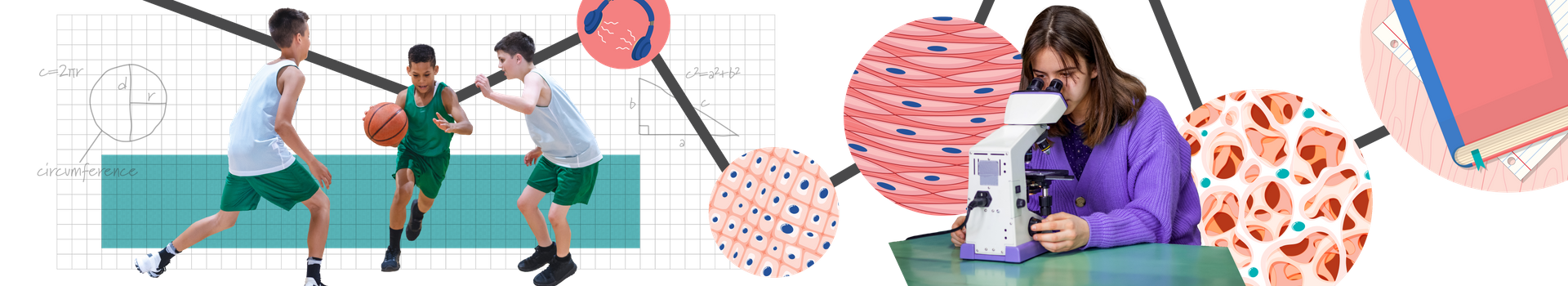In Grade 8 Mathematics, our students will complete their intermediate math journey by exploring extremely large and extremely small number using scientific notation and solving problems relating to area, perimeter, volume, and angles. Their understanding of operations will extend to include whole numbers, fractions, decimals, percentages, and negative numbers (integers). They will also continue exploring patterns and representing patterns using algebra and the analysis and representation of data and probability. Their financial literacy will be consolidated by analyzing budgets and setting and maintaining financial goals. In coding, our students will apply data analysis within the code to user-inputted information to assist in informing decisions. This course is designed to be 200-300 instructional hours.

Curriculum Information: Mathematics (2020)

Developed by: D2L

Development Date: 2020

Please note that elementary courses do not have prerequisites, and Ontario schools do not issue transcript credits at the elementary level. All courses are available in the facilitated and independent pathways.

Facilitated

Independent

#### Course Outline

Representing Numbers

In the Representing Numbers unit, students learn how to represent large and small numbers including using scientific notation. Students also learn how to describe, compare, and order rational and irrational numbers.

Geometry

In the Geometry unit, students solve problems involving area, perimeter, circumference, area, volume, and angles. Students are introduced to the Pythagorean Theory as a way to find missing side lengths of right triangles. Students develop their understanding about scale drawings, transformations of shapes, and tessellation. Students also learn about large and small units of measurement.

Operations

In the Operations unit, students solve single-step, multi-step, and multi-operation problems involving rational numbers and percentages, and extend their knowledge to multiply and divide integers. Student develop mental math strategies to divide whole numbers and decimal numbers by powers of ten. In addition, students explore square numbers and square roots.

Fractions

In the Fractions unit, students extend their learning to add, subtract, divide, and multiply fractions and mixed numbers.

Proportional Reasoning

In the Proportional Reasoning unit, students solve single-step, multi-step, and multi-operation problems involving fractions, decimal numbers, ratios, rates, and percentages.

Data

In the Data unit, students extend their knowledge to represent, analyse, and describe relationships about data. Students also develop their understanding of probability.

Patterning and Algebra

In the Patterning and Algebra unit, students learn to determine the general term of a pattern using algebraic expression, create their own patterns, and describe them in different ways. Student solve algebraic equations in different ways and learn about inequalities represented with algebra.

Coding

In the Coding unit, students learn to read, create, alter, and debug code that involves data analysis and use that analysis to inform decisions.

Measurement

In the Measurement unit, students solve problems involving perimeter, circumference, area, surface area, and volume of 2D and 3D shapes.

Financial Literacy

In the Financial Literacy unit, students learn how to create and maintain a balanced budget to reach a financial goal. In addition, they analyse simple and compound interest, credit cards, and reward programs that give them the best value for their money.

Socio-Emotional Learning

Throughout the course, students build their social emotional learning skills by specifically focusing on relationships with others, using math to communicate and tell a story, and understanding the perspectives of others. This increases their understanding of common bonds with peers and their appreciation of the uniqueness of other people.

#### Required Resources

This course is entirely online and does not require nor rely on any textbook. Students will require the following resources:

• A scanner, smartphone camera, or similar device to digitize handwritten or hand-drawn work
• A device to record audio
• A printer
• A physical binder, folder, or notebook for offline activities
• Calculator
• Protractor
• Compass
• Ruler with centimetres
• Various household items to complete offline activities

#### Teaching and Learning Strategies

Through a balance of problem-solving and direct instruction, students develop a strong foundation of mathematical processes, knowledge, and skills to apply in real-world contexts. The course engages multiple learning styles by combining technology and offline activities that provide opportunities to develop an understanding of skills and concepts in interactive and concrete ways. The lessons feature a variety of intriguing storylines, videos, graphics, and interactive games to reinforce students’ learning. The activities also build a foundation of mathematical models and strategies that students will use throughout their elementary grades.

The course relies on the assistance of a learning coach who supports young students as they move through the content. The learning coach will be involved in facilitating technical aspects of the course and in participating in discussion-based activities to assist students in developing communication skills.